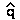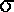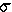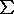Mutation, Migration, Inbreeding, & Genetic Drift in natural populations

How do mutation, migration, inbreeding, and genetic drift interact with selection? Do they maintain or reduce variation?
Can they maintain variation at a high level?
What is their significance in population (short-term) & evolutionary (long-term) biology?

(1) Mutation / selection equilibrium

Deleterious alleles are maintained by recurrent mutation.
A stable equilibrium(whereq = 0) is reached
when the rate of replacement (by mutation)
balances the rate of removal (by selection).

µ = frequency of new mutant alleles per locus per generation
typical µ = 10-6: 1 in 1,000,000 gametes has new mutant
_____
then=(µ / s)      [see derivation]

Ex.: For a recessive lethal allele (= 1) with a mutation rate of µ = 10-6
thenû(10-6 / 1.0) = 0.001

Lowering selection against alleles increases their frequency.
Medical intervention has increased the frequency of heritable conditions
in Homo (e.g., diabetes, myopia)
Eugenics: modification of human condition by selective breeding
'positive eugenics': encouraging people with "good genes" to breed
'negative eugenics': discouraging people with 'bad genes'' from breeding
e.g., immigration control, compulsory sterilization
[See: S. J. Gould, "The Mismeasure of Man"]

Is eugenics effective at reducing frequency of deleterious alleles?
What proportion of 'deleterious alleles' are found in heterozygous carriers?

(2pq) / 2q2 = p/q1/q    (if  q << 1)

if s = 1 as above, ratio is 1000 / 1 : most of variation is in heterozygotes,
not subject to selection

(2) Migration / selection equilibrium

Directional selection is balanced by influx of 'immigrant' alleles;
a stable 'equilibrium' can be reached iff migration rate constant.

Consider an island adjacent to a mainland, with unidirectional migration to the island.
The fitness values of the AA, AB, and BB genotypes differ in the two environments,
so that the allele frequencies differ between the mainland (qm) and the island (qi).

 AA AB BB W0 W1 W2 q Island 1 1-t 1-2t qi0 Mainland 0 0 1 qm1

B has high fitness on mainland, and low fitness on island.
[For this model only, allele A is semi-dominant to allele B,
so we use t for the selection coefficient to avoid confusion]

m = freq. of new migrants (with qm) as fraction of residents (with qi)
if m << t    qi = (m / t)(qm)      [see derivation]

Gene flow can hinder optimal adaptation of a population to local conditions.

Ex: Water snakes (Natrix sipedon) live on islands in Lake Erie (Camin & Ehrlich 1958)
Island Natrix mostly unbanded; on adjacent mainland, all banded.
Banded snakes are non-cryptic on limestone islands, eaten by gulls
Suppose   A = unbanded     B = banded   [AB are intermediate]
Let      qm = 1.0     ["B" allele is fixed on mainland]
m   = 0.05   [5% of island snakes are new migrants]
t    = 0.5     so  W2 = 0  ["Banded" trait is lethal on island]
then    qi = (0.05/0.5)(1) = 0.05
and     Hexp = 2pq = (2)(0.95)(0.05)10%
i.e, about 10% of snakes show intermediate banding, despite strong selection

=> Recurrent migration can maintain a disadvantageous trait at high frequency.

(3) Inbreeding / selection

Inbreeding is the mating of (close) relatives
or, mating of individuals with at least one common ancestor

F (Inbreeding Coefficient) = prob. of "identity by descent":
Expectation that two alleles in an individual are
exact genetic copies of an allele in the common ancestor

or, proportion of population with two alleles identical by descent

This is determined by the consanguinity (relatedness) of parents.

Inbreeding reduces Hexp  by a proportion F
(& increases the proportion of homozygotes). [see derivation]

f(AB) = 2pq (1-F)
f(BB) = q2 + Fpq
f(AA) = p2 + Fpq

Inbreeding affects genotype proportions,
inbreeding does not affect allele frequencies.

Inbreeding increases the frequency of individuals
with deleterious recessive genetic diseases by F/q [see derivation]

Ex.: if q = 10-3 and F = 0.10 , F/q = 100
=> 100-fold increase in f(BB) births

Inbreeding coefficient of a population can be estimated from experimental data:

F =  ( 2pq - Hobs ) / 2pq  [see derivation]

Ex.: Selander (1970) studied structure of Mus house mice living in chicken sheds in Texas

 obs exp AA 0.226 0.181 AB 0.400 0.489 BB 0.374 0.329
since p = 0.226 + (1/2)(0.400) = 0.426

&   q = 0.374 + (1/2)(0.400) = 0.574

Then F = (0.489 - 0.400) / (0.489) = 0.182
which is intermediate between Ffull-sib      = 0.250
&  F1st-cousin = 0.125

=> Mice live in small family groups with close inbreeding
[This is typical for small mammals]

Inbreeding is usually thought of as "harmful":
inbreeding increase the probability that deleterious recessive alleles
will come together in homozygous combinations
"Harmful" alleles are reinforced
Inbreeding depression: a loss of fitness in the short-term due to
difficulty in conception, increased spontaneous abortion, pre- & peri-natal deaths
Ex.: First-cousin marriages in Homo
Two-fold increase in spontaneous abortion & infant mortality
Every human carries 3 ~ 4 "lethal equivalents"

Demonsration #2: Selection & inbreeding in small populations

However, in combination with natural selection, inbreeding can be "advantageous":
increases rate of evolution in the long-term (q0 more quickly)
deleterious alleles are eliminated more quickly.
increases phenotypic variance (homozygotes are more common).
advantageous alleles are also reinforced in homozygous form

(4) Genetic Drift / selection

Genetic Drift is stochasticq [unpredictable, random]
(cf. deterministicq [predictable, due to selection, mutation, migration)

Sewall Wright (1889 - 1989): "Evolution and the Genetics of Populations"

Stochasticq  > deterministicq in small populations:
allele frequencies drift more rapidly in 'small' than 'large' populations.

Drift is most noticeable if0, and/or N small (< 10)  [1/s]
genetic varianceq2 = (q)(1 - q) / 2N

if q = p = 0.5, thenq2 = 1 / 8N

<>       q drifts between generations (within population variance decreases) [DEMO];

eventually, allele is lost (q = 0) or fixed (q = 1) (if qi = 0.5, 50:50 odds)

q drifts among populations (among population variance increases);
eventually, half lose the allele, half fix it.

Ex [Demonstration #3]
[Try: q = 0.5, W0 = W1 = W2 = 1.0, and N = 10, 50, 200, 1000;
repeat 10 trials each, note q at endpoint
]

**=> Variation is 'fixed' or 'lost'  &  populations will diverge by chance <=**

Evolutionary significance:
"Gambler's Dilemma" : if you play long enough, you win or lose everything.
All populations are finite: many are very small, somewhere or sometime.
Evolution occurs on vast time scales: "one in a million chance" is a certainty.
Reproductive success of individuals in variable: "The race is not to the swift ..."

What happens in the really long run?

Effective Population Size (Ne)
= size of an 'ideal' population with same genetic variation (measured as H)
as the observed 'real' population.
= The 'real' population behaves evolutionarily like one of size Ne :
e.g., the population will drift like one of size Ne
loosely the number of breeding individuals in the population

Consider three special cases where Ne < or << Nobs  [the 'count' of individuals]:

(1) Unequal sex ratio

Ne = (4)(Nm)(Nf) / (Nm  + Nf)

where Nm & Nf are numbers of breeding males & females, respectively.

"harem" structures in mammals (Nm << Nf)
Ex.: if Nm  = 1 "alpha male" and  Nf = 200
then     Ne = (4)(1)(200)/(1 + 200)4
A single male elephant seal (Mirounga) does most of the breeding
Elephant seals have very low genetic variation

eusocial (colonial) insects like ant & bees (Nf << Nm)
Ex.: if Nf = 1 "queen" and  Nm= 1,000 drones
then     Ne = (4)(1)(1,000)/(1 + 1,000)4
Hives are like single small families

(2) Unequal reproductive success
In stable population, Noffspring/parent = 1
"Random" reproduction follows Poisson distribution (N = 11)
(some parents have 0, most have 1, some have 2, a few have 3 or more)

 XNe = Reproductive strategy 1 1 Nobs Breeding success is random 1 0 2 x Nobs A zoo-breeding strategy 1 >1 < Nobs K-strategy, as in Homo 1 >>1 << Nobs r-strategy, as in Gadus

(3) Population size variation over time

Ne = harmonic mean of N  = inverse of arithmetic mean of inverses
[a harmonic mean is much closer to lowest value in series]
n
Ne = n /  [(1/Ni) ]  where Ni = pop size in i th generation
i=1

Populations exist in changing environments:
Populations are unlikely to be stable over very long periods of time
10-2 forest fire / 10-3 flood / 10-4 ice age

Ex.: if typical N = 1,000,000  & every 100th generation N = 10 :
then  Ne = (100) / [(99)(10-6) + (1)(1/10)]100 / 0.1 = 1,000

Founder Effect & Bottlenecks:
Populations are started by (very) small number of individuals  (N=2),
or undergo dramatic reduction in size (N < 10)).

Ex.: Origin of Newfoundland moose (Alces):
2 bulls + 2 cows at Howley in 1904
[maximum 8 alleles / locus, likely less if single source population]
[1 bull + 1 cow at Gander in 1878 didn't succeed].

Population cycles: Hudson Bay Co. trapping records (Elton 1925)
Population densities of lynx, hare, muskrat cycle over several orders of magnitude
Lynx cycle appears to "chase" hare cycle

The effect of drift on genetic variation in populations

Larger populations are more variable (higher H) than smaller
if s = 0H reflects balance between loss of alleles by drift
and replacement by mutation

H = (4Neµ) / (4Neµ + 1) [the "neutralist equation"]

Ex.: if µ= 10-7   & Ne = 106   then   Neµ = 1   and Hexp = (0.4)/(0.4 + 1) = 0.29

But typical Hobs0.20  which suggests  Ne105
Most natural populations have a much smaller effective size than their typically observed size.

Ex.: Gadus morhua in W. Atlantic were confined to Flemish Cap during last Ice Age 8~10 KYBP
mtDNA sequence variation occurs as "star phylogeny":
most variants are rare and related to a common surviving genotype
Carr et al. 1995 estimated Ne = 3x104  as compared with Nob s109
Effective size is ca. 5 orders of magnitude smaller than observed

Marshall et al. (2004) showed that "genome-types" at Flemish Cap are distinctive

Stochastic effects may be as or more important than deterministic processes in long-term evolution.

Text material © 2004 by Steven M. Carr